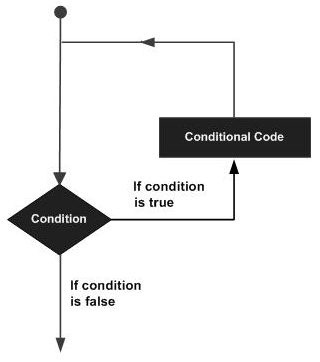# Rexx - Loops

So far we have seen statements which have been executed one after the other in a sequential manner. Additionally, statements are provided in Rexx to alter the flow of control in a program’s logic. They are then classified into a flow of control statements which we will study in detail.

A loop statement allows us to execute a statement or group of statements multiple times. The following illustration is the general form of a loop statement in most of the programming languages.Let us discuss various loops supported by Rexx.

Sr.No. Loop Type & Description
1 do loop

The do loop is used to execute a number of statements for a certain number of times. The number of times that the statement needs to be executed is determined by the value passed to the do loop.

2 do-while loop

The do-while statement is used to simulate the simple while loop which is present in other programming languages.

3 do-until loop

The do-until loop is a slight variation of the do while loop. This loop varies in the fact that is exits when the condition being evaluated is false.

## Controlled Repetition

The do loops can be catered to carry out a controlled repetition of statements.

### Syntax

The general syntax of this sort of statement is as follows.

```do index = start [to limit] [by increment] [for count]
statement #1
statement #2
end
```

The difference in this statement is that there is an index which is used to control the number of times the loop is executed. Secondly, there are parameters which state the value which the index should start with, where it should end and what is the increment value.

### Flow Diagram

Let’s check out the flow diagram of this loop −From the above diagram you can clearly see that the loop is executed based on the index value and how the index value is incremented.

The following program is an example of the controlled repetition statement.

### Example

```/* Main program */
do i = 0 to 5 by 2
say "hello"
end
```

In the above program, the value of the count i is set to 0 first. Then it is incremented in counts of 2 till the value is not greater than 5.

The output of the above code will be −

```hello
hello
hello
```